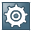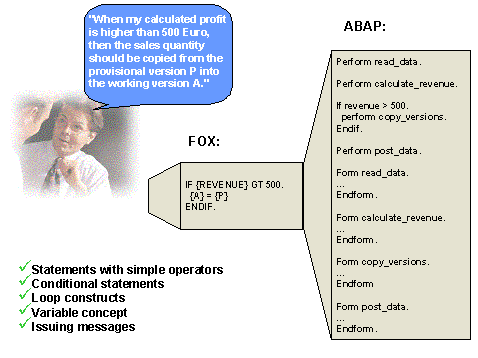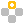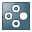##Formula### Use

You can define formulas which define how the transaction data is to be processed in order to generate plan data. Formula functions enable you to use extended mathematical functions to calculate plan data.

In addition to different calculation functions, which you can use for value assignment in formulas, there is also the possibility to model complex flow structures with the formula language FOX. In this way you get closer to the possibilities that you have when using exit functions. However, the complexity of the programming is considerably less for formulas than for ABAP statements which you have to program when using user exits. The following graphic illustrates this difference by comparing the solution of a business planning task with the methods of FOX and of ABAP:### Integration

If, in the source code of a formula, you create connections between data records (for example, “increase planned profit for region EMEA by 10%, when the profit made in region Asia exceeds 5 million Dollars; otherwise by 5%”), you need to know about data transfer to planning functions in BW-BPS.

### Features

When executing a formula function, the values of key figures in a planning level are always changed.

You have to specify those fields that are to be changed during the calculation as fields to be changed. These fields are for planning level characteristics and the Key Figure Name field.  Field selection allows you to define whether the transformation described by the formula should take place for all key figures in the same way, or only for certain key figures.

The following different types of formula calculation exist:

·        For the fields to be changed, select characteristics only and not the generic entry key figure name. The transformation is performed for all the key figures of a planning level. It is not necessary to formulate a separate equation for all key figures.If you select the characteristic fiscal year, the following formula, for example, can be formed: { 2004 } = { 2003 } * 1.1.

The calculation is performed for all key figures for the year 2004.Remarks on syntax: If characteristic values appear in operands, operands have to be enclosed in curly brackets { and } so as to avoid operands being confused with constants.

For the formula calculation, the values of the characteristic that you are using for addressing are not restricted to the values in the package.This is how formula Plan = 1.15 * actual, for example, works even if the package is restricted to data of type Plan, data of type Actual is read automatically too.If you select the field Key Figure Name for the conditions, you can restrict calculation to key figures of your own choice. The key figures that you explicitly assign a value to, in the definition of a parameter group in the formula editor, are then transformed.

·        Include the generic entry Key Figure Name and one or more characteristics in the fields to be changed. In this case for every operand in the formula editor, you specify a combination of one value for every characteristic included in the list and if applicable the name of the key figure to be changed.If you select the field Key Figure Name as the field to be changed and any characteristic, for example, fiscal year, the following formula is, for example, formed: { REVENUE, 2004 } =  { PRICE, 2003 } * { SQTY,  2003 } * 1.1.

The key figure is only calculated for the year 2004.  The data for 2003 that is consulted for the calculation does not have to be in the package or level.

·        Select the generic entry Key Figure Name as the field to be changed for the formula function and select particular characteristics as the condition fields of a revaluation function. The transformation is only performed for data records for which the affected characteristic value combination exists.  When creating a parameter group for the function, a separate formula editor is displayed for every one of these characteristics. You can enter the formula for the respective characteristic value combination in this formula editor.If you only select the Key Figure Name as the field to be changed, the following formula, for example, is formed:
REVENUE = PRICE * SQTY

The formula is calculated for each individual record that meets the conditions.

You can find more information on how the selection of characteristics and key figures affect operand syntax in the formula editor under Formula Operands (Dependent on Key Figure and Characteristic Selection).

#### Mathematical Operators and Functions

You can use the following mathematical functions and operators to calculate a key figure:

·        Single-figure operators

+, (as debit/credit signs)

·        Two-figure operators

+, , *, /, DIV (division in whole numbers), MOD (modulo division), ** (multiplying)

·        Investment mathematical functions

DECL (straight-line depreciation), DECD (declining-balance method of depreciation), DISC (discounting), PERP (perpetual bond)

·        Other functions

ABS, CEIL, FLOOR, FRAC, TRUNC, SIGN, MAX, MIN, SQRT, LOG, LOG10, EXP, COS, SIN,TAN, COSH, TANH, ASIN, ACOS, ATAN

You find further information (in particular about the number and meaning of function parameters) in the F1 help.

#### Processing Several Formulas in a Formula Function

All functions return a simple score (and no time series).

However in special cases, for example with depreciation functions, you want to observe the development of the net book value in chronological order. You can attain this by including both the generic entry Key Figure Name as well as a time-bearing characteristic, for example fiscal year, in the list of fields to be changed. The structure of the operands arises from this { <Key figure name>, FYEAR }.

You can process a number of formulas in one step using your own formula function by entering the required formulas in succession in the formula editor.For a complete straight-line depreciation of a acquisition worth 10,000 € with a yearly depreciation rate of 25% (corresponding to a duration of depreciation of 4 years), beginning in the year 2000, you enter the following formula in the formula editor:
{ DEPREC, 2003 } = DECL ( 10000, 0, 25, 1 )
{ DEPREC, 2004 } = DECL ( 10000, 0, 25, 2 )
{ DEPREC, 2005 } = DECL ( 10000, 0, 25, 3 )
{ DEPREC, 2006 } = DECL ( 10000, 0, 25, 4 )

As a result, every formula delivers the net book value of the acquisition after the specified number of years.

You can carry out calculations for numerous key figures simultaneously within one parameter group in the formula editor. Here interim results from prior formula steps within a parameter group are referred to for further calculations in this parameter group.The following examples show how you can use key figures in the formula editor in different ways:

REVENUE = PRICE * SALES QUANTITY
ROCE = NOPAT / CAPEMPL

The key figures do not overlap – every key figure appears exactly once in the quantity of all formulas in the formula editor.

REVENUE = REVENUE * 1.1
ROCE = NOPAT / CAPEMPL

The key figure REVENUE appears on both sides of the assignment operator.

PRICE = PRODUCTION COSTS * 1.2
REVENUE = PRICE * SALES QUANTITY

The key figure PRICE appears in different formulas on different sides of the assignment operator; PRICE goes into the second formula with the value that resulted as the interim result from the calculation in the first formula. The following sample values should make this clearer for the different execution periods.

 Key figure Before execution After 1st formula After 2nd formula PRICE 7 9,6 9,6 PRODUCTION COSTS 8 8 8 REVENUE 0 0 960 SALES QUANTITY 100 100 100

#### Reusing Parameter Groups

The parameter groups for a formula function can be quite comprehensive and execute complex operations. BW-BPS offers you the possibility to transfer the formula code of any other parameter group into a parameter group created by you.  All parameter groups of formula functions existing in the system are available for this.

To transfer the code of another parameter group, proceed as follows:

...

1.      Create a new parameter group for a formula function.

2.      ChooseCopy Formula.

The system displays all existing parameter groups for formula functions in a list.

3.      Select the parameter group which you want to transfer and confirm your selection.

The system inserts the formula statements of the parameter group you selected into the formula editor of the new parameter group.